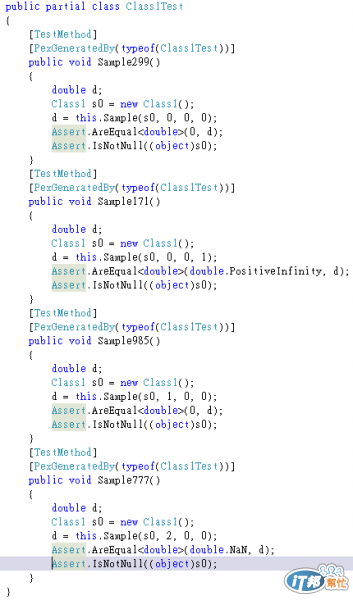#DAY 24
5

## [如何提升系統品質-Day24]測試 - Code Coverage

[如何提升系統品質]系列文章連結
Code Coverage的定義

Code Coverage的種類
Code Coverage種類不少，以下舉出幾種：
1.function coverage：

2.Line Coverage：

3.Statement Coverage：

4.Decision Coverage：

``````        public double Sample(double a, double b, double x)
{

if (a > 0 && b == 0)
{
x = x / a;
}

if (a == 2 || x == 1)
{
x = x / b;
}

return x;
}
``````

statement coverage要100%，test case只需要a=2, b=0, x=0，即每一個statement都會跑到。但一樣每一個statement都測到了，就代表這個測試完美了嗎？no, 因為判斷式的條件有多個，判斷式裡面的陳述式所回傳的bool值，可能發生意想不到的錯誤。

5.Condition Coverage：

``````if a and b then
``````

a=true, b=false
a=false, b=true

6.Condition/Decision Coverage：

7.Modified condition/decision coverage：

``````if (a or b) and c then
``````

a=true, b=true, c=true
a=false, b=false, c=false

a=false, b=false, c=true => 第一個陳述式為false，第二個陳述式為true，and結果為false
a=true, b=false, c=true => 第一個陳述式為true，第二個陳述式為true，and結果為true
a=false, b=true, c=true => 第一個陳述式為true，第二個陳述式為true，and結果為true
a=true, b=true, c=false => 第一個陳述式為true，第二個陳述式為false，and結果為false

8.Multiple Condition Coverage：

a=false, b=false, c=false
a=false, b=false, c=true
a=false, b=true, c=false
a=false, b=true, c=true
a=true, b=false, c=false
a=true, b=false, c=true
a=true, b=true, c=false
a=true, b=true, c=true

Code Coverage的工具

Tools for C# .NET
DevPartner
JetBrains dotCover
Kalistick
NCover
TestDriven.NET
Visual Studio 2010

Microsoft Research有出一個很棒的東西叫做Pex and Moles，可以讓你很『快速』的產生高程式碼覆蓋率的單元測試程式，且支援多種Unit Test Framework。這邊的快速，指的是可以省去我們思考不同的test case滿足不同路徑的時間，但Pex還是需要時間、CPU去計算出滿足所有路徑的input/output。Code Coverage的迷思

1.有沒有做單元測試？
2.重要的Scenario有沒有涵蓋多一點情境？
3.現在有哪些程式碼沒有被測過？
4.發生測試失敗時，失敗的原因點production code是否有被test case涵蓋到。